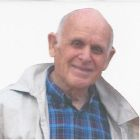Categories Pricing CorporateFree Textbook

# Essential Mathematics for Engineers

0 Reviews
268
Language:  English
This book is written for engineers and students and covers a range of mathematics from school to university. The first chapter is a summary so students can quickly see where further study is needed.
Content
Description

1. Arithmetic
2. Algebra
3. Geometry
4. Trigonometry
5. Co-ordinate Geometry
6. Logorithms
7. Permutations and Combinations
8. Matrices and Determinants
9. Series
10. Calculus
11. Numerical Solution of Equation
12. Expansion into a Series
13. Hyperbolic Functions
14. Methods for Integration
15. Functions of Time and Other Variables
16. Areas and Volumes
17. Maxima and Minima
18. Graphs
19. Vectors
20. Argand Diagram
21. Differential Equations
22. Bessell’s and Legendre’s Equations
23. Laplace Transform
24. Fourier Series
25. Mechanics’ Elementary Principles
26. Rotational Motion
27. Forces Acting on a Body
28. Simple Harmonic Motion (or SHM)
29. Structures
30. Hanging Chains
31. Gyroscopes

Elementary Mathematics for Engineers explains mathematical jargon and develops the formulae used by engineers from first principles. The first chapter is a summary so the reader can quickly see where further study is needed. The book is in two parts, pure and applied. Pure mathematics covers the following subjects;

• positive, negative, and fractional indices
• logarithms
• partial fractions
• irrational and complex functions
• trigonometrical and hyperbolic functions
• properties of triangles and polygons
• areas and volumes
• rules for differentiation
• methods for integration
• differential equations
• Fourier analysis
• Applied mathematics covers the following subjects;
• forces in equilibrium
• equations of motion and Newton’s laws
• potential and kinetic energy
• conservation of energy
• momentum and angular momentum
• simple harmonic motion
• hanging chains
• gyroscopes

W. J. R. H. Pooler

ONC, MA (Cantab) class 1, CENG, MIEE, MIMechE

I studied for and obtained Ordinary National Certificate while working as an apprentice at the English Electric Co, Stafford. This included time in their high voltage laboratory. I then went to Cambridge University and passed the Mechanical Sciences Tripos after two years with First Class Honours. For the third year, I carried out further studies on heavy electrical power machines. After graduating, I joined the Iraq Petroleum Company in Kirkuk, Iraq and was later appointed Protection Engineer and System Control Engineer responsible for the operation of the high voltage network and for the hands on commissioning of all new electrical plant including 66kv and 11kv cables and lines and transformers up to 5MVA and motors up to 2000 hp.

I was then appointed Head of Electrical Engineering at Basrah Petroleum Co responsible for four power stations, 33kv and 11kv transformers, cables and lines and motors up to 1500 hp. The operation of all Instrumentation in the Production Plants and all Telecommunications in the Company was later added to my responsibilities.

This book is based on experiences gained during this period.

You can contact me on john.pooler@tiscali.co.uk